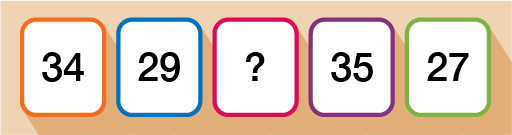Science, Maths & Technology

### Become an OU studentEveryday maths 2

Start this free course now. Just create an account and sign in. Enrol and complete the course for a free statement of participation or digital badge if available.

# 6.5 Calculating missing data when given the mean

Sometimes, rather than being given the data and asked to find the mean, you may be given some of the data as well as the mean and asked to find the missing piece of data.

## Case study _unit4.6.1 Example: Finding the missing number 1Figure _unit4.6.3 Figure 21 Calculating missing data using the mean

The mean of the numbers on 4 cards is 15. Find the missing number.

### Method

If you think about how we work out the mean – add up all the numbers and then divide by how many numbers there are – for this example, the calculation looks like this:

#### Extract _unit4.6.1

= 15

If we ‘undo’ the division by 4, by multiplying both sides by 4 we are left with:

#### Extract _unit4.6.2

Total of numbers = 15 × 4

Total of numbers = 60

Now that you know the total of all 4 numbers is 60, to find the missing number you can simply subtract the numbers that you do know from 60.

#### Extract _unit4.6.3

60 – 14 – 20 – 16 = 10

The missing number is therefore 10.

Let’s take a look at a slightly different example.

## Case study _unit4.6.2 Example: Finding the missing number 2

A rugby team has played a total of 12 matches over the season. The mean average number of points scored is 14. The team play one more match and score 20 points. How does this affect the mean average over the 13 games?

### Method

Again, you know that for this example:

#### Extract _unit4.6.4

= 14

Similarly to the last example, if you ‘undo’ the division by 12 by multiplying both sides by 12, you are left with:

#### Extract _unit4.6.5

Total of numbers = 12 × 14

Total of numbers = 168

Now you know the total of points scored in the first 12 games played. You have been told in the question that the team play one more game and score 20 points. The new total for all 13 games is therefore 168 + 20 = 188 points.

To find the mean we now do, = 14.5 (rounded to one d.p.). Therefore, the mean average increases after the thirteenth game is played.

Now have a go at the activity below and check your knowledge and understanding.

## Activity _unit4.6.4 Activity 12: Problem solving using the mean

1. The mean of all 5 cards is 31. Work out the missing number.Figure _unit4.6.4 Figure 22 Problem solving using the mean
1. A shop works out the mean average sales over the first 6 months of the year. The mean for these 6 months is £2400. In the following month, the mean increases to £2500. How much did the shop make in the seventh month?

1. = 31

Total of numbers = 31 × 5

Total of numbers = 155

Now you know the total of all 5 cards, do:

• 155 – 34 – 29 – 35 – 27 = 30

The missing number is 30.

2. For this question you first need to work out the total money made by the shop in the first 6 months:

• = £2400

Total of numbers = £2400 × 6

• Total of numbers = £14,400

• Now you know this, you can work out the total amount of money raised by the shop over the 7-month period:

•  = £2500

Total of numbers = £2500 × 7

Total of numbers = £17,500

• To work out the amount raised in the seventh month, now do £17,500 − £14,400 = £3100 raised in the seventh month.